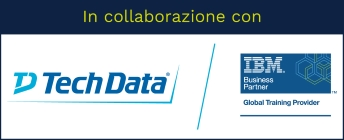Stai utilizzando un browser vecchio, che non rispetta gli standard web. Ti consigliamo di cambiarlo, perché questo sito potrebbe non funzionare correttamente.

# Predictive Modeling for Continuous Targets Using IBM SPSS Modeler (v18.1.1)

## IBM0A0V8G

This course provides an overview of how to use IBM SPSS Modeler to predict a target field that describes numeric values. Students will be exposed to rule induction models such as CHAID and C&R Tree. They will also be introduced to traditional statistical models such as Linear Regression. Students are introduced to machine learning models, such as Neural Networks. Business use case examples include: predicting the length of subscription for newspapers, telecommunication, and job length, as well as predicting insurance claim amounts.

### Argomenti

1: Introduction to predicting continuous targets

• List three modeling objectives
• List two business questions that involve predicting continuous targets
• Explain the concept of field measurement level and its implications for selecting a modeling technique
• List three types of models to predict continuous targets
• Determine the classification model to use
2: Building decision trees interactively
• Explain how CHAID grows a tree
• Explain how C&R Tree grows a tree
• Build CHAID and C&R Tree models interactively
• Evaluate models for continuous targets
• Use the model nugget to score records
3: Building your tree directly
• Explain the difference between CHAID and Exhaustive CHAID
• Explain boosting and bagging
• Identify how C&R Tree prunes decision trees
• List two differences between CHAID and C&R Tree
4: Using traditional statistical models
• Explain key concepts for Linear
• Customize options in the Linear node
• Explain key concepts for Cox
• Customize options in the Cox node
5: Using machine learning models
• Explain key concepts for Neural Net
• Customize one option in the Neural Net node

### Obiettivi

1: Introduction to predictive models for continuous targets

• List three modeling objectives
• List two business questions that involve predicting continuous targets
• Explain the concept of field measurement level and its implications for selecting a modeling technique
• List three types of models to predict continuous targets
• Determine the classification model to use

2: Building decision trees interactively

• Explain how CHAID grows a tree
• Explain how C&R Tree grows a tree
• Build CHAID and C&R Tree models interactively
• Evaluate models for continuous targets
• Use the model nugget to score records

3: Building decision trees directly

• Customize two options in the CHAID node
• Customize two options in the C&R Tree node
• List one difference between CHAID and C&R Tree
1. Using traditional statistical models
• Explain key concepts for Linear
• Customize options in the Linear node
• Explain key concepts for Cox
• Customize options in the Cox node

5: Using machine learning models

• Explain key concepts for Neural Net
• Customize one option in the Neural Net node

## Prezzo di listino

700,00 EUR + IVA per partecipante

• 8 ore
• 1 giorno

## Prerequisiti

• Experience using IBM SPSS Modeler including familiarity with the Modeler environment, creating streams, reading data files, exploring data, setting the unit of analysis, combining datasets, deriving and reclassifying fields, and a basic knowledge of modeling.
• Prior completion of Introduction to IBM SPSS Modeler and Data Science (v18.1.1) is recommended.

13 luglio

11 ottobre

# Erogabile on-line e on-site

Tutti i nostri corsi sono erogabili anche in modalità on-line (con formazione a distanza), oppure on-site, sempre personalizzati secondo le esigenze.